## 4.7Total pressure condition

Sec. 4.6 concluded that the basic outﬂow conditions —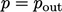and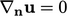— are generally unstable for a free boundary with both inﬂow and outﬂow.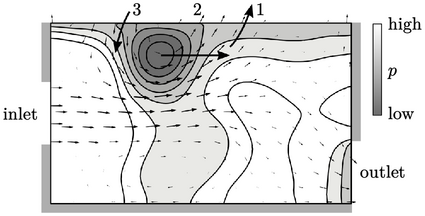The conditions are unstable due to pressure ﬂuctuations at the boundary, shown above. Flows oscillates in and out, shown by 3 stages, creating a vortex that travels from left to right:

1. the pressure gradient, decreasing outward, causes outﬂow;
2. the outﬂow speed increases causing the pressure gradient to change direction;
3. inﬂow begins and the speed increases, until the pressure gradient changes direction, returning back to step 1.

The total pressure boundary condition improves the stability of solutions. It is a ﬁxed value type, calculated according to: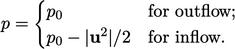(4.7)
The speciﬁed total pressure,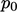, can be imagined as the ﬂuid pressure under quiescent conditions far from the free boundary, which decreases as the ﬂuid accelerates towards the boundary. Note that Eq. (4.7 ) is written for the incompressible assumption, Eq. (2.47 ), where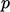andare kinematic, i.e. divided by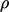.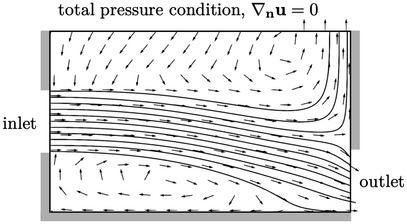The solution using the total pressure condition converges to the ﬂow ﬁeld shown above. The critical eﬀect of this boundary condition is that, the boundarydecreases by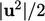as the inﬂow speed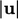increases. This reduces the pressure gradient driving inﬂow, which moderates the increase in inﬂow speed, enabling it to settle to a stable level.

### Total pressure for high speed ﬂow

The total pressure condition can be applied to high-speed ﬂow of a compressible gas. The calculation offor inﬂow is simply replaced with the 1D isentropic ﬂow equation,4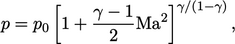(4.8)
where Mach number is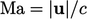and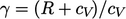.
4Ascher Shapiro, The dynamics and thermodynamics of compressible ﬂuid ﬂow, Vol. 1, Ch. 4, 1953.

Notes on CFD: General Principles - 4.7 Total pressure condition